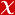## RESOLUTION OF THE EQUATION (3(x1)-1)(3(x2)-1) = (5(y1)-1)(5(y2)-1)

ROCKY MOUNTAIN JOURNAL OF MATHEMATICS, vol.50, no.4, pp.1425-1433, 2020 (Journal Indexed in SCI)• Publication Type: Article / Article
• Volume: 50 Issue: 4
• Publication Date: 2020
• Doi Number: 10.1216/rmj.2020.50.1425
• Title of Journal : ROCKY MOUNTAIN JOURNAL OF MATHEMATICS
• Page Numbers: pp.1425-1433

#### Abstract

Consider the diophantine equation (3(x1) - 1)(3(x2) - 1) = (5(y1) - 1)(5(y2) - 1) in positive integers x(1) <= x(2) and y(1) <= y(2). Each side of the equation is a product of two terms of a given binary recurrence. We prove that the only solution to the title equation is (x(1), x(2), y(1), y(2)) = (1, 2, 1, 1). The main novelty of our result is that we allow products of two terms on both sides.